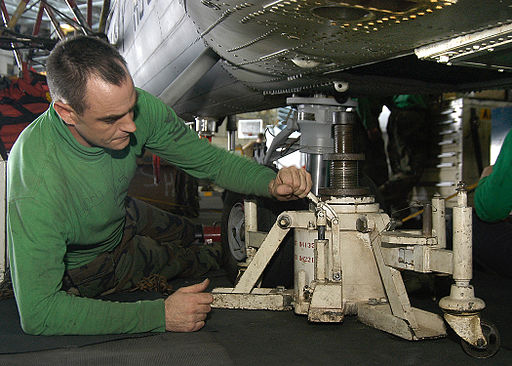# Difference Between Force and Pressure

## Main Difference – Force vs. Pressure

In everyday usage, the terms force and pressure may have similar connotations. However, the scientific usage of these terms are not the same. The main difference between force and pressure is that force is described as an interaction which has the ability cause acceleration while pressure is defined as the force acting perpendicular to a surface per unit area.

## What is Force

From Newton’s second law, we can describe a force as an interaction which has the ability to cause acceleration. Of course, if the net force on an object is 0 then there would not be a net acceleration. More simply, forces are often described as pushes or pulls.

A force is needed to make a stationary body move, or to speed up, slow down or change the direction of motion of a body that is already in motion. Force is a vector quantity with a specified direction, measured in units of newtons (N).

## What is Pressure

Pressure$p$ is the magnitude of force$F$ acting perpendicular to a surface per unit area:$p=\frac{F}{A}$

The surface of a body immersed in fluid experiences a pressure due to the weight of fluid above it. Here, the orientation of the surface does not matter. Pressure always acts perpendicular to the surface.

We experience atmospheric pressure all the time, from the weight of air above us. Blood pressure exerted from inside our body keeps us from imploding under the atmospheric pressure. A diver diving in deep see experiences a pressure equal to the atmospheric pressure plus pressure from the weight of water above them. In order to counter the additional pressure, pressure suits are worn.

Note that two surfaces with different areas immersed at the same depth in a fluid experiences the same pressure but different forces. A related principle is used to work hydraulic jacks. Here, the same pressure is transferred along an enclosed body of fluid from one end of a container to the other. By having different sizes of pistons at each end, the force given from one piston can be amplified at the other end.The difference between force and pressure is exemplified in the working principle of a hydraulic jack. At one end of the jack, the user exerts a smaller force on the smaller piston. There is a fluid within the jack, which transmits the same pressure to the other end of the jack. At that end, the piston size is larger, which causes an increased force to act on the car.

Pressure is a scalar quantity and it is measured in units of pascals (Pa). 1 Pa = 1 N m-2. Often, units of atmospheres (atm) are also used to measure pressure. (1 atm = 101325 Pa).

## What is the difference between Force and Pressure

### Definition

Force is an interaction which has the ability to cause an acceleration.

Pressure is the force exerted perpendicular to a surface per unit area.

### Nature of Quantity

Force is a vector quantity.

Pressure is a scalar quantity.

### Units

Force is measured in newtons (N).

Pressure is measured in pascals (Pa).

Image Courtesy

“Arabian Gulf (June 6, 2004) – Aviation Machinist’s Mate 2nd Class Robert Henry of Lewiston, Minn., adjusts a hydraulic jack…” by U.S. Navy photo by Photographer’s Mate Airman Robert Brooks (United States Navy with the ID 040606-N-9630B-009) [Public Domain], via Wikimedia Commons